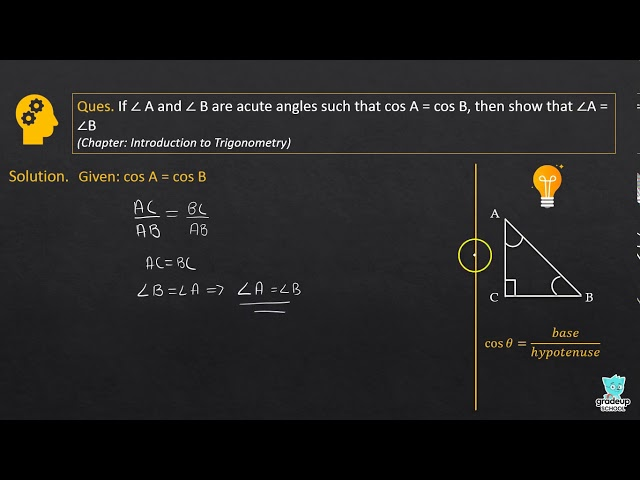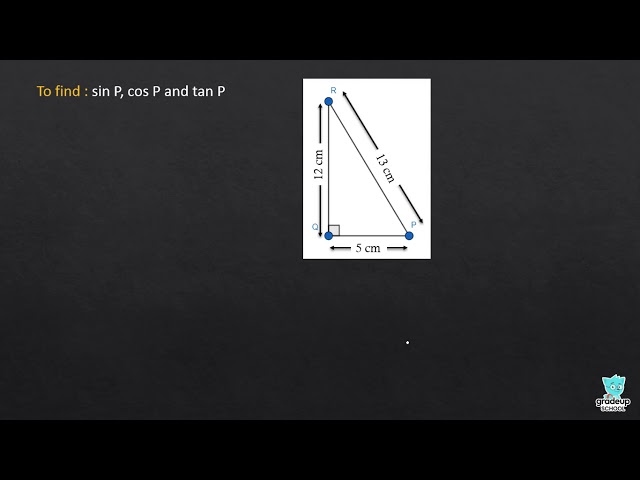# NCERT Solutions for Class 10 Maths Chapter 8 - Introduction to Trigonometry

Share

NCERT Mathematics Solutions for Class 10t Chapter 8 - Introduction to Trigonometry is prepared by an experienced team of subject mentors of Goprep to help students in solving the questions of the NCERT textbook of Class 10. The Solutions given to all the questions are provided with a brief explanation and step-by-step so that students can clear their doubts and make a strong foundation of the topics in an efficient way.

Maths is a subject that demands in-depth understanding and reasoning skills in order to excel in the subject. So, with the help of these NCERT Solutions,  you can clear your concepts of the topic in a simple and easy manner. In this Chapter, you will study Trigonometric Ratios of the Angle, Acute Angle, Trigonometric Identities, Trigonometric Ratios of Complementary Angles, Trigonometric Ratios of Some Specific Angles and many such topics in an organized format.

## NCERT Solutions for Class 10 Maths Chapter 8 - Introduction to Trigonometry

Exercise 8.1
• Exercise 8.1
• Exercise 8.2
• Exercise 8.3
• Exercise 8.4
• Testing
Class 10th|NCERT - MathematicsChapter 8 - Introduction to TrigonometrySee More (1)
NCERT - MathematicsClass 10th , NCERT
 Chapter 1 - Real Numbers Chapter 2 - Polynomials Chapter 3 - Pair of Linear Equations in Two Variables Chapter 4 - Quadratic Equations Chapter 5 - Arithmetic Progressions Chapter 6 - Triangles Chapter 7 - Coordinate Geometry Chapter 8 - Introduction to Trigonometry Chapter 9 - Some Applications of Trigonometry Chapter 10 - Circles Chapter 11 - Constructions Chapter 12 - Areas Related to Circles Chapter 13 - Surface Areas and Volumes Chapter 14 - Statistics Chapter 15 - Probability